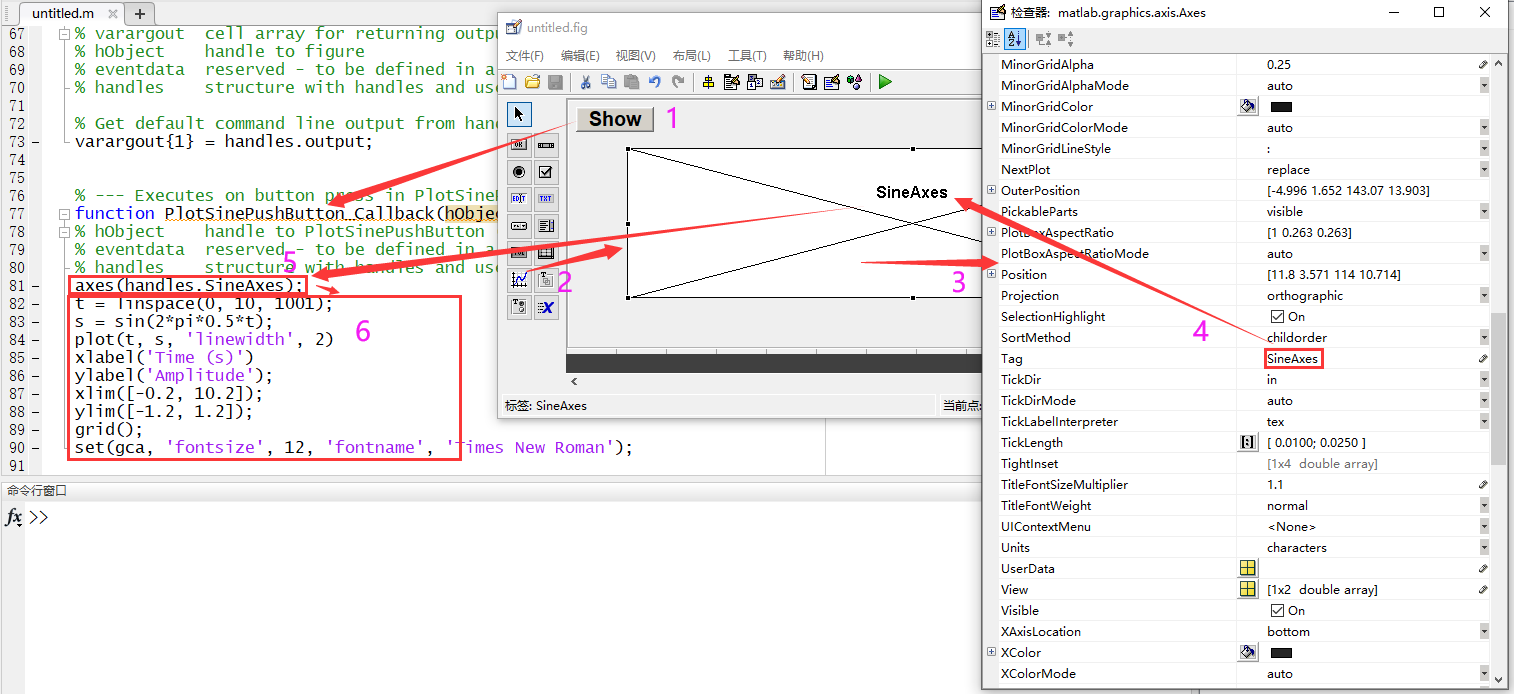### 1 Add an axes on the GUI app

We introduced how to add a button on a MATLAB GUI app in the previous part. Here we will show that how to plot the graph of a sine function on the app, and we suppose that you have created a guide file. We need to add an axes on the app before we implement plotting a sine function.

• 1 Add a button (see the previous part);
• 3 Double-click on the axes to call the axes setting interface;
• 4 Set the axes name;
• 5 Go and implement the callback function of the button;
• 6 The specific codes of plotting a sine function.

One can note that we need to get the axes before we plot the sine function, and the code is

axes( handles.SineAxes);.### 2 Run this app and plot a sine function

We need run the MATLAB GUI file if we want to plot the sine function.

• 1 Run untitled.m;
• 2 Press Show button;
• 3 The sine function will be shown.Adding an axes to plot is very simple, isn’t it?